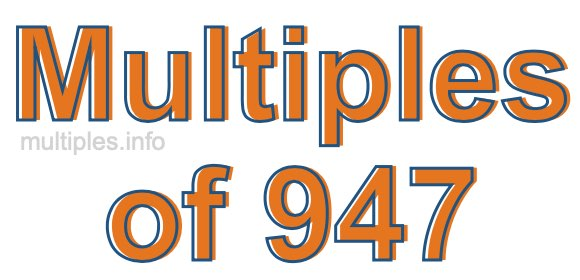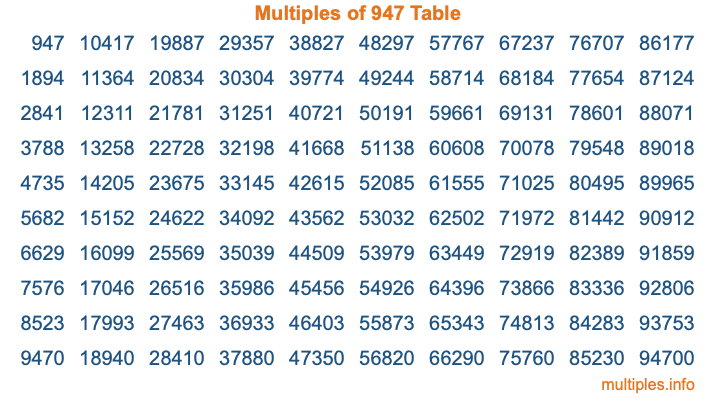Multiples of 947Welcome to the Multiples of 947 page. Here we will first teach you everything you will ever need to know about the multiples of 947, and then give you a study guide summary of everything we taught you to make sure you remember it all. Use this page to look up facts and learn information about the multiples of 947. This page will make you a multiples of nine hundred forty-seven expert!

Definition of Multiples of 947
Multiples of 947 are all the numbers that when divided by 947 equal an integer. Each of the multiples of 947 are called a multiple. A multiple of 947 is created by multiplying 947 by an integer.

Therefore, to create a list of multiples of 947, you start with 1 multiplied by 947, then 2 multiplied by 947, then 3 multiplied by 947, and so on for as long as you want. Thus, the list of the first five multiples of 947 is 947, 1894, 2841, 3788, and 4735. To see a larger list of multiples of 947, see the printable image of Multiples of 947 further down on this page. We also have a category where you can choose any nth multiple of 947.

Multiples of 947 Checker
The Multiples of 947 Checker below checks to see if any number of your choice is a multiple of 947. In other words, it checks to see if there is any number (integer) that when multiplied by 947 will equal your number. To do that, we divide your number by 947. If the the quotient is an integer, then your number is a multiple of 947.

Is  a multiple of 947?

Least Common Multiple of 947 and ...
A Least Common Multiple (LCM) is the lowest multiple that two or more numbers have in common. This is also called the smallest common multiple or lowest common multiple and is useful to know when you are adding our subtracting fractions. Enter one or more numbers below (947 is already entered) to find the LCM.

Check out our LCM Calculator if you need more details about the Least Common Multiple or if you need the LCM for different numbers for adding and subtraction fractions.

nth Multiple of 947
As we stated above, 947 is the first multiple of 947, 1894 is the second multiple of 947, 2841 is the third multiple of 947, and so on. Enter a number below to find the nth multiple of 947.

th multiple of 947

Multiples of 947 vs Factors of 947
947 is a multiple of 947 and a factor of 947, but that is where the similarities end. All postive multiples of 947 are 947 or greater than 947. All positive factors of 947 are 947 or less than 947.

Below is the beginning list of multiples of 947 and the factors of 947 so you can compare:

Multiples of 947: 947, 1894, 2841, 3788, 4735, etc.

Factors of 947: 1, 947

As you can see, the multiples of 947 are all the numbers that you can divide by 947 to get a whole number. The factors of 947, on the other hand, are all the whole numbers that you can multiply by another whole number to get 947.

It's also interesting to note that if a number (x) is a factor of 947, then 947 will also be a multiple of that number (x).

Multiples of 947 vs Divisors of 947
The divisors of 947 are all the integers that 947 can be divided by evenly. Below is a list of the divisors of 947.

Divisors of 947: 1, 947

The interesting thing to note here is that if you take any multiple of 947 and divide it by a divisor of 947, you will see that the quotient is an integer.

Multiples of 947 Table
Below is an image of the first 100 multiples of 947 in a table. The table is in chronological order, column by column. The first column has the first ten multiples of 947, the second column has the next ten multiples of 947, and so on.The Multiples of 947 Table is also referred to as the 947 Times Table or Times Table of 947. You are welcome to print out our table for your studies.

Negative Multiples of 947
Although not often discussed or needed in math, it is worth mentioning that you can make a list of negative multiples of 947 by multiplying 947 by -1, then by -2, then by -3, and so on, to get the following list of negative multiples of 947:

-947, -1894, -2841, -3788, -4735, etc.

Multiples of 947 Summary
Below is a summary of important Multiples of 947 facts that we have discussed on this page. To retain the knowledge on this page, we recommend that you read through the summary and explain to yourself or a study partner why they hold true.

There are an infinite number of multiples of 947.

A multiple of 947 divided by 947 will equal a whole number.

947 divided by a factor of 947 equals a divisor of 947.

The nth multiple of 947 is n times 947.

The largest factor of 947 is equal to the first positive multiple of 947.

947 is a multiple of every factor of 947.

947 is a multiple of 947.

A multiple of 947 divided by a divisor of 947 equals an integer.

947 divided by a divisor of 947 equals a factor of 947.

Any integer times 947 will equal a multiple of 947.

Multiples of a Number
Here you can get the multiples of another number, all with the same attention to detail as we did for multiples of 947 on this page.

Multiples of
Multiples of 948
Did you find our page about multiples of nine hundred forty-seven educational? Do you want more knowledge? Check out the multiples of the next number on our list!